# Define symmetric matrix with the help of an example

Define symmetric matrix with the help of an example.

A square matrix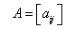is said to be symmetric if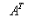= A, that is,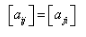For all possible values of i and j.
For example: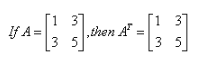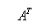= A